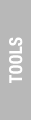#### Tool Selection

The Mass-Volume Conversion Calculator calculates a substance's mass of a given volume relative to its compaction dynamics. It is still common to find volumetrically measured ingredients, even where by weight is deemed more accurate; or ingredients measured by weight when a scale is not available. In these cases, this tool provides an accurate way to convert from one method of measurement to the other, due to the compensation for a granular substance's tendency to compact under its own weight. Some common incompressible liquids have also been added for your convenience.

The Mixed Mass Percentage Calculator calculates deaggregated mass quantities relative to elemental percentages, given a target mass and a target elemental percentage. It is sometimes necessary to combine two products together to achieve the qualities of a missing product. Attaining a flour with a certain percentage of protein is a very common example, and one that this tool is suited to help determine the proportions for.

The Precision Perfect Calculator calculates ideal totals to which given percentages can be applied for the purpose of increasing accuracy based on limited precision of measurement devices.

The Hydration Calculator calculates the moisture density in air based on a given temperature and relative humidity, as well as the hydration absorption of a flour according to its composition.

#### Mass-Volume Conversion Calculator

Substance

Measurement Method

The Textbook method is recommended to provide consistent and reliable results when measuring flour. It involves stirring the flour in the container to loosen, repeatedly lifting the flour from the container into a measuring cup, and leveling off the flour in the cup with a flat edge.
The Light option is both an alternative method of measurement and a compaction cancellation setting. Use this option when sifting flour into the measuring cup through a mesh sieve or if the effects of compaction are to be ignored. This option is only recommended for flour.
The Medium option represents common practices which result in more compaction than would be expected using the Textbook method. These practices include dipping the measuring cup into the flour or vibrating the measuring cup of flour. The ×2 option indicates using both practices.
The Heavy option is an alternative method of measurement. It involves transferring the flour from the container into a measuring cup, striking the cup on the work surface, repeating the process until the flour has fully settled, and leveling off the flour in the cup with a flat edge.

Measurement Conversion

Mass → Volume Volume → Mass

Enter the total mass of the substance into the Total field after choosing the desired unit of measure and deselecting unavailable cups.
 ```Total gram ounce ``` ` cup(s)` ` 1/3 cup(s)` ` 3/4 cup(s)` ` 1/4 cup(s)` ` 2/3 cup(s)` ` tablespoon(s)` ` 1/2 cup(s)` ` teaspoon(s)`

#### Mixed Mass Percentage Calculator

Choose substance A or enter a percentage:

`  %`

Choose substance B or enter a percentage:

`  %`

Enter the target mass and percentage:

 `Mass ` ` % → Mass Mass → %` ` ` ` %` `MassA` `MassB`